#### Thank you for registering.

One of our academic counsellors will contact you within 1 working day.

Please check your email for login details.

Click to Chat

1800-1023-196

+91-120-4616500

CART 0

• 0
MY CART (5)

Use Coupon: CART20 and get 20% off on all online Study Material

ITEM
DETAILS
MRP
DISCOUNT
FINAL PRICE
Total Price: Rs.

There are no items in this cart.
Continue Shopping• Complete JEE Main/Advanced Course and Test Series
• Offered Price: Rs. 15,900
• View Details

# Chapter 14: Coordinate Geometry Exercise – 14.3

### Question: 1

Find the coordinates of the point which divides the line segment joining (-1, 3) and (4, - 7) internally in the ratio 3 : 4.

### Solution:

Let P(x, y) be the required point.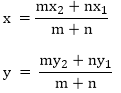Here, x1 = - 1

y1 = 3

x= 4

y2 = -7

m : n = 3 : 4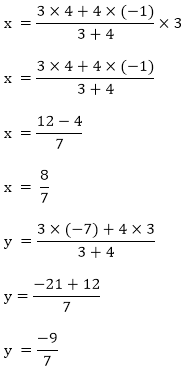∴ The coordinates of P are (8/7, – 9/7)

### Question: 2

Find the points of trisection of the line segment joining the points:

(i) (5, - 6) and (-7, 5)

(ii) (3, - 2) and (-3, - 4)

(iii) (2, - 2) and (-7, 4)

### Solution:

(i) Let P and Q be the point of trisection of AB i.e., AP = PQ = QBTherefore. P divides AB internally in the ratio of 1: 2, thereby applying section formula, the coordinates of P will beNow, Q also divides AB internally in the ratio of 2:1 there its coordinates are(ii) Let P, Q be the point of tri section of AB i.e. ,

AP = PQ = QB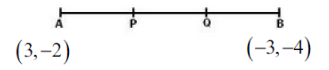Therefore, P divides AB internally in the ratio of 1: 2. Hence by applying section formula. Coordinates of P are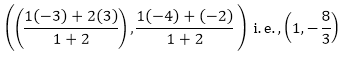Now, Q also divides as internally in the ratio of 2: 1

So, the coordinates of Q areLet P and Q be the points of trisection of AB i.e., AP = PQ = OQTherefore, P divides AB internally in the ratio 1:2. Therefore, the coordinates of P, by applying the section formula, are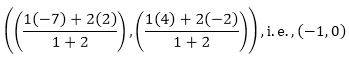Now. Q also divides AB internally in the ration 2 : 1. So the coordinates of Q are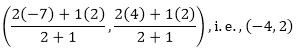### Question: 3

Find the coordinates of the point where the diagonals of the parallelogram formed by joining the points (-2, -1), (1, 0), (4, 3) and (1, 2) meet.

### Solution: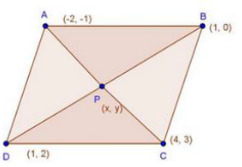Let P(x, y) be the given points.

We know that diagonals of a parallelogram bisect each other.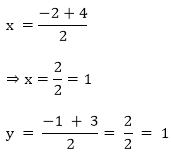∴  coordinates of P are (1, 1)

### Question: 4

Prove that the points (3, 2), (4, 0), (6, -3) and (5, -5) are the vertices of a parallelogram.

### Solution: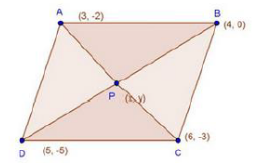Let P(x, y) be the point of intersection of diagonals AC and 80 of ABCD.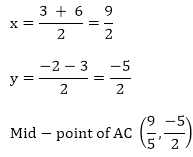Again,Here mid-point of AC — Mid - point of BD i.e. diagonals AC and BD bisect each other.

We know that diagonals of a parallelogram bisect each other

∴ ABCD is a parallelogram.

### Question: 5

Three consecutive vertices of a parallelogram are (- 2, -1), (1, 0) and (4, 3). Find the fourth vertex.

### Solution:

Let A (-2,—1), B (1, 0), C (4, 3)and D (x, y) be the vertices of a parallelogram ABCD taken in order.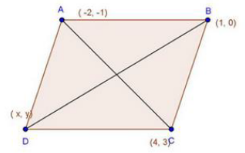Since the diagonals of a parallelogram bisect each other.

∴ Coordinates of the mid - point of AC = Coordinates of the mid-point of BD.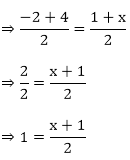⇒ x + 1 = 2

⇒ x = 1

And,Hence, fourth vertex of the parallelogram is (1, 2)## Complete JEE Main/Advanced Course and Test Series

OFFERED PRICE: Rs. 15,900
View Details Xpress Buy

### Course Features

• 731 Video Lectures
• Revision Notes
• Test paper with Video Solution
• Mind Map
• Study Planner
• NCERT Solutions
• Discussion Forum
• Previous Year Papers

### Course Features

• 728 Video Lectures
• Revision Notes
• Previous Year Papers
• Mind Map
• Study Planner
• NCERT Solutions
• Discussion Forum
• Test paper with Video Solution